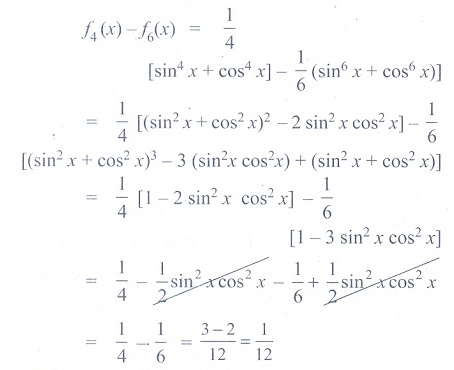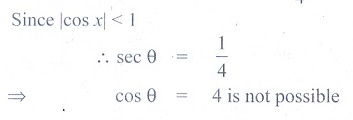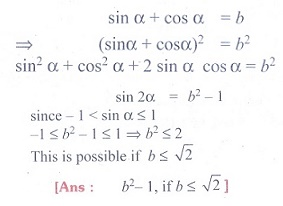Home | | Maths 11th std | Exercise 3.12: Choose the correct or the most suitable answer

# Exercise 3.12: Choose the correct or the most suitable answer

Multiple choice questions with answers / choose the correct answer with answers - Maths Book back 1 mark questions and answers with solution for Exercise Problems - Mathematics : Trigonometry

CHAPTER : Trigonometry

1. 1/cos 80╠Ŗ  - ŌłÜ3/sin 80╠Ŗ =

(1) ŌłÜ2

(2) ŌłÜ3

(3) 2

(4) 4

Solution2. If cos 28╠Ŗ  + sin 28╠Ŗ  = k3, then cos 17╠Ŗ  is equal to

(1) k3/ŌłÜ2

(2) -k3/ŌłÜ2

(3) ┬▒ k3/ŌłÜ2

(4) - k3/ŌłÜ2

Solution3. The maximum value of 4 sin2x + 3cos2x + sin x/2 + cos x/2 is

(1) 4 + ŌłÜ2

(2) 3 + ŌłÜ2

(3) 9

(4) 4

Solution4.(1) 1/8

(2)1/2

(3)1/ŌłÜ3

(4)1/ŌłÜ2

Solution5. If, equal to

(1) -2cos╬Ė

(2) -2sin╬Ė

(3) 2cos╬Ė

(4) 2sin╬Ė

Solution (Option : (4) 2sin╬Ė or (1) -2cos╬Ė ??????? Please don't refer this solution for examination)6. If tan40╠Ŗ = ╬╗, then(1) 1-╬╗2/ ╬╗

(2) 1+╬╗2/ ╬╗

(3) 1+╬╗2/ 2╬╗

(4) 1-╬╗2/ 2╬╗

Solution7. cos 1╠Ŗ + cos 2╠Ŗ + cos 3╠Ŗ + ŌĆ”. + cos 179╠Ŗ =

(1) 0

(2) 1

(3) -1

(4) 89

Solution8. Let fk(x) =1/k [sinkx + coskx] where x ŌłŖ R and k Ōēź 1. Then f4(x) - f6(x) =

(1)1/4

(2)1/12

(3)1/6

(4)1/3

Solution9. Which of the following is not true?

(1) sin ╬Ė = -3/4

(2) cos ╬Ė = -1

(3) tan ╬Ė = 25

(4) sec ╬Ė =1/4

Solution10. cos2╬Ė cos2ŽĢ + sin2 (╬Ė - ŽĢ) - sin2 (╬Ė + ŽĢ) is equal to

(1) sin 2(╬Ė + ŽĢ)

(2) cos 2(╬Ė + ŽĢ)

(3) sin 2(╬Ė - ŽĢ)

(4) cos 2(╬Ė - ŽĢ)

Solution11.(1) sinA + sinB + sinC

(2) 1

(3) 0

(4) cosA + cosB + cosC

Solution12. If cos p╬Ė + cos q╬Ė = 0 and if p ŌēĀ q, then ╬Ė is equal to (n is any integer)Ans: 2

Solution13.If tan╬▒ and tan╬▓ are the roots of x2 + ax + b = 0, thenis equal to

(1)b/a

(2)a/b

(3) ŌĆōa/b

(4) ŌĆōb/a

Solution14. In a triangle ABC, sin2A + sin2B + sin2C = 2, then the triangle is

(1) equilateral triangle

(2) isosceles triangle

(3) right triangle

(4) scalene triangle.

Solution15. If f(╬Ė) = |sin ╬Ė| + |cos╬Ė| , ╬Ė ŌłŖ R, then f(╬Ė) is in the interval

(1) [0, 2]

(2) [1,ŌłÜ2]

(3) [1, 2]

(4) [0, 1]

Solution16. cos 6x + 6 cos 4x + 15 cos 2x + 10 / cos 5x + 5 cos 3x + 10 cos x is equal to

(1) cos2x

(2) cos x

(3) cos 3x

(4) 2 cos x

Solution17. The triangle of maximum area with constant perimeter 12m

(1) is an equilateral triangle with side 4m

(2) is an isosceles triangle with sides 2m, 5m, 5m

(3) is a triangle with sides 3m, 4m, 5m

(4) Does not exist.

Solution18. A wheel is spinning at 2 radians/second. How many seconds will it take to make 10 complete rotations?

(1) 10ŽĆ seconds

(2) 20 ŽĆ seconds

(3) 5 ŽĆ seconds

(4) 15ŽĆ seconds

Solution19. If sin╬▒ + cos╬▒ = b, then sin 2╬▒  is equal to

(1) b2 - 1, if b Ōēż ŌłÜ2

(2) b2 - 1, if b > ŌłÜ2

(3) b2 - 1, if b Ōēź 1

(4) b2 - 1, if b ŌēźŌłÜ2

Solution20. In a ŌłåABC, if

(i) sin A/2 sin B/2 sin C/2> 0

(ii) sinAsinB sinC > 0 then

(1) Both (i) and (ii) are true

(2) Only (i) is true

(3) Only (ii) is true

(4) Neither (i) nor (ii) is true.

SolutionTags : Trigonometry | Mathematics , 11th Mathematics : UNIT 3 : Trigonometry
Study Material, Lecturing Notes, Assignment, Reference, Wiki description explanation, brief detail
11th Mathematics : UNIT 3 : Trigonometry : Exercise 3.12: Choose the correct or the most suitable answer | Trigonometry | Mathematics